## Incenter

The centerof a Triangle's Incircle. It can be found as the intersection of Angle Bisectors, and it is the interior point for which distances to the sidelines are equal. Its Trilinear Coordinates are 1:1:1. The distance between the incenter and Circumcenter is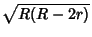.

The incenter lies on the Euler Line only for an Isosceles Triangle. It does, however, lie on the Soddy Line. For an Equilateral Triangle, the Circumcenter, Centroid, Nine-Point Center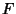, Orthocenter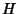, and de Longchamps Pointall coincide with.

The incenter and Excenters of a Triangle are an Orthocentric System. The Power of the incenter with respect to the Circumcircle is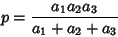(Johnson 1929, p. 190). If the incenters of the Triangles,, andare,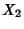, and, thenis equal and parallel to, whereare the Feet of the Altitudes andare the incenters of the Triangles. Furthermore,,,, are the reflections ofwith respect to the sides of the Triangle(Johnson 1929, p. 193).

If four points are on a Circle (i.e., they are Concyclic), the incenters of the four Triangles form a Rectangle whose sides are parallel to the lines connecting the middle points of opposite arcs. Furthermore, the connectors pass through the center of the Rectangle (Fuhrmann 1890, p. 50; Johnson 1929, pp. 254-255). More generally, the 16 incenters and excenters of the Triangles whose Vertices are four points on a Circle, are the intersections of two sets of four Parallel lines which are mutually Perpendicular (Johnson 1929, p. 255).

References

Carr, G. S. Formulas and Theorems in Pure Mathematics, 2nd ed. New York: Chelsea, p. 622, 1970.

Dixon, R. Mathographics. New York: Dover, p. 58, 1991.

Fuhrmann, W. Synthetische Beweise Planimetrischer Sätze. Berlin, 1890.

Johnson, R. A. Modern Geometry: An Elementary Treatise on the Geometry of the Triangle and the Circle. Boston, MA: Houghton Mifflin, pp. 182-194, 1929.

Kimberling, C. Central Points and Central Lines in the Plane of a Triangle.'' Math. Mag. 67, 163-187, 1994.

Kimberling, C. Incenter.'' http://cedar.evansville.edu/~ck6/tcenters/class/incenter.html.